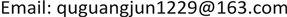﻿ 一类非线性椭圆型边界值问题的正解 Positive Solutions for a Class of Nonlinear Elliptic Boundary Value Problem

Advances in Applied Mathematics
Vol.07 No.07(2018), Article ID:25986,6 pages
10.12677/AAM.2018.77103

Positive Solutions for a Class of Nonlinear Elliptic Boundary Value Problem

Guangjun Qu

School of Mathematics and Computer Science, Shaanxi University of Technology, Hanzhong ShaanxiReceived: Jun. 24th, 2018; accepted: Jul. 13th, 2018; published: Jul. 20th, 2018ABSTRACT

Using the Ekeland’s variantional principle and a variant version mountain pass lemma, two positive solutions are obtained for a class of nonlinear elliptic boundary value problem.

Keywords:Ekeland’s Variantional Principle, Variant Version Mountain Pass Lemma, Positive SolutionsCopyright © 2018 by author and Hans Publishers Inc.

This work is licensed under the Creative Commons Attribution International License (CC BY).

http://creativecommons.org/licenses/by/4.0/1. 引言

$\left\{\begin{array}{ll}-{\Delta }_{p}u=\lambda f\left(x,u\right),\hfill & x\in \Omega \text{\hspace{0.17em}}.\hfill \\ u=0,\hfill & x\in \partial \Omega \text{\hspace{0.17em}}.\hfill \end{array}$ (1)

f1) $\underset{t\to {0}^{+}}{\mathrm{lim}}\frac{f\left(x,t\right)}{{t}^{p-1}}=+\infty$ a.e. $x\in \stackrel{¯}{\Omega }$

f2) $\underset{t\to +\infty }{\mathrm{lim}}\frac{f\left(x,t\right)}{{t}^{p-1}}=+\infty$ a.e. $x\in \stackrel{¯}{\Omega }$

f3) 存在常数 $\theta \ge 1,{\theta }_{0}>0$ 使得 $\theta G\left(x,s\right)\ge G\left(x,t\right)-{\theta }_{0}$ $\forall x\in \stackrel{¯}{\Omega }$$0\le t\le s$ 都成立。其中， $G\left(x,t\right)=f\left(x,t\right)t-pF\left(x,t\right)$$F\left(x,t\right)={\int }_{0}^{t}f\left(x,s\right)\text{d}s$

f4) 当 $n>p$ 时， $\exists q\in \left(p,\frac{np}{n-p}\right)$ ；当 $n\le p$ 时， $\exists q\in \left(p,+\infty \right)$ ，s. t. $\underset{t\to +\infty }{\mathrm{lim}}\frac{f\left(x,t\right)}{{t}^{q-1}}=0$ a.e. $x\in \stackrel{¯}{\Omega }$

f5) 当 $t\ge 0$$x\in \stackrel{¯}{\Omega }$ 时， $f\left(x,t\right)\ge 0$ ；当 $t\le 0$$x\in \stackrel{¯}{\Omega }$ 时， $f\left(x,t\right)\equiv 0$

f2') $\underset{t\to +\infty }{\mathrm{lim}}\frac{f\left(x,t\right)}{{t}^{p-1}}=l$ a.e. $x\in \stackrel{¯}{\Omega }$ ，其中l是常数。

$f\left(x,t\right)$ 满足条件(f2')，则称 $f\left(x,t\right)$ 在无穷远处是渐近线性的；若 $f\left(x,t\right)$ 满足条件(f2)，则称 $f\left(x,t\right)$ 在无穷远处是超线性的；很明显(f2)和(f2')是不相容的。

f1') ${b}_{0}\le \underset{t\to {0}^{+}}{\mathrm{lim}}\mathrm{inf}\frac{f\left(x,t\right)}{{t}^{p-1}}\le \underset{t\to {0}^{+}}{\mathrm{lim}}\mathrm{sup}\frac{f\left(x,t\right)}{{t}^{p-1}}\le a\left(x\right)$ a. e. $x\in \stackrel{¯}{\Omega }$ ，其中 ${b}_{0}$ 为常数， $a\left(x\right)\in {L}^{\infty }\left(\Omega \right)$ 满足

$\forall x\in \stackrel{¯}{\Omega }$ ，都有 $a\left(x\right)\le {\lambda }_{1}$ ，且存在某正测集 ${\Omega }_{1}\subset \Omega$ 使得 $a\left(x\right)\le {\lambda }_{1}$ a. e. $x\in \stackrel{¯}{\Omega }$${\lambda }_{1}$$-{\Delta }_{p}$ 的第一个特征值。

2. 预备知识

$\left(1+‖{u}_{k}‖\right){I}^{\prime }\left({u}_{k}\right)\to 0\text{\hspace{0.17em}}\left(k\to \infty \right)$

$\mathrm{max}\left\{I\left(0\right),I\left(e\right)\right\}\le \alpha <\beta \le \underset{‖u‖=\rho }{\mathrm{inf}}I\left(u\right)$

$c=\underset{\gamma \in \Gamma }{\mathrm{inf}}\underset{0\le t\le 1}{\mathrm{max}}I\left(\gamma \left(t\right)\right)$ ，其中 $\Gamma =\left\{\gamma \in C\left(\left[0,1\right],E\right):\gamma \left(0\right)=0,\gamma \left(1\right)=e\right\}$ 为连结0与e的道路的集合。则存

$I\left({u}_{n}\right)\to c\ge \beta$ ，且 $\left(1+‖{u}_{n}‖\right){‖{I}^{\prime }\left({u}_{n}\right)‖}_{{E}^{\ast }}\to 0\text{\hspace{0.17em}}\left(n\to \infty \right)$

3. 主要结果及其证明

${J}_{\lambda }\left(u\right)=\frac{1}{p}{\int }_{\Omega }{|\nabla u|}^{p}\text{d}x-\lambda {\int }_{\Omega }F\left(x,u\right)\text{d}x$$\forall u\in {W}_{0}^{1,p}\left(\Omega \right)$

${J}_{\lambda }\in {C}^{1}\left({W}_{0}^{1,p}\left(\Omega \right),R\right)$ ，且寻找方程(1)的非平凡解等价于寻找泛函 ${J}_{\lambda }$ 的非零临界点。

$f\left(x,t\right)\le {c}_{1}{|t|}^{q-1},\forall \left(x,t\right)\in \stackrel{¯}{\Omega }×R$

$F\left(x,t\right)\le \frac{{c}_{1}}{q}{|t|}^{q},\forall \left(x,t\right)\in \stackrel{¯}{\Omega }×R$(2)

${J}_{\lambda }\left(u\right)=\frac{1}{p}{‖u‖}^{p}-\lambda {\int }_{\Omega }F\left(x,u\right)\text{d}x\ge \frac{1}{p}{‖u‖}^{p}-\lambda {\int }_{\Omega }\frac{{c}_{1}}{q}{|u|}^{q}\text{d}x\ge \frac{1}{p}{‖u‖}^{p}-\lambda {c}_{2}{‖u‖}^{q}$ (3)

$\beta =\frac{1}{p}{\rho }^{p}-\lambda {c}_{2}{\rho }^{q}>0$

$f\left(x,t\right)\ge \frac{{t}^{p-1}}{\epsilon }-m$$\forall \left(x,t\right)\in \stackrel{¯}{\Omega }×R$

$F\left(x,t\right)\ge \frac{1}{p\epsilon }{t}^{p}-mt$$\forall \left(x,t\right)\in \stackrel{¯}{\Omega }×R$

${\varphi }_{1}>0\left(‖{\varphi }_{1}‖=1\right)$${\lambda }_{1}$ 对应的正则特征函数，则

${\int }_{\Omega }\frac{F\left(x,t{\varphi }_{1}\right)}{{t}^{p}}\text{d}x\ge {\int }_{\Omega }\left(\frac{1}{p\epsilon }{\varphi }_{1}^{p}-\frac{m{\varphi }_{1}}{{t}^{p-1}}\right)\text{d}x$ . (4)

$\underset{t\to +\infty }{\mathrm{lim}}\mathrm{inf}{\int }_{\Omega }\frac{F\left(x,t{\varphi }_{1}\right)}{{t}^{p}}\text{d}x\ge {\int }_{\Omega }\frac{1}{p\epsilon }{\varphi }_{1}^{p}\text{d}x$

$\epsilon >0$ 是任意的，故当 $\epsilon \to {0}^{+}$ 时，可得

$\underset{t\to +\infty }{\mathrm{lim}}{\int }_{\Omega }\frac{F\left(x,t{\varphi }_{1}\right)}{{t}^{p}}\text{d}x=+\infty$

$\frac{{J}_{\lambda }\left(t{\varphi }_{1}\right)}{{t}^{p}}=\frac{1}{p}-{\int }_{\Omega }\frac{F\left(x,t{\varphi }_{1}\right)}{{t}^{p}}\text{d}x\to -\infty \left(t\to +\infty \right)$

${J}_{\lambda }\left({u}_{n}\right)\to c$ ，且 $\left(1+‖{u}_{n}‖\right)‖{{J}^{\prime }}_{\lambda }\left({u}_{n}\right)‖\to 0\left(n\to \infty \right)$ . (5)

$\frac{1}{p}〈{{J}^{\prime }}_{\lambda }\left({u}_{n}\right),{u}_{n}〉=o\left(1\right)$ ，从而

${\int }_{\Omega }\left(\frac{\lambda }{p}f\left(x,{u}_{n}\right){u}_{n}-\lambda F\left(x,{u}_{n}\right)\right)\text{d}x=c+o\left(1\right)$ . (6)

${W}_{n}\stackrel{弱}{\to }W$${W}_{0}{}^{1,p}\left(\Omega \right)$ 中；有 ${W}_{n}\stackrel{强}{\to }W$${L}^{q}\left(\Omega \right)$ 中； ${W}_{n}\left(x\right)\to W\left(x\right)$ a.e. $x\in \Omega$ . (7)

${W}^{+}\equiv 0$ 。选取一个实数序列 $\left\{{t}_{n}\right\}$ ，使得 ${J}_{\lambda }\left({t}_{n}{u}_{n}\right)=\underset{t\in \left[0,1\right]}{\mathrm{max}}{J}_{\lambda }\left(t{u}_{n}\right)$ 。对任意的正整数k，定义 ${V}_{n}={\left(2pk\right)}^{\frac{1}{p}}{W}_{n}{}^{+}$ ，因为 ${W}^{+}\equiv 0$ ，则

$\underset{n\to \infty }{\mathrm{lim}}{\int }_{\Omega }F\left(x,{V}_{n}\right)\text{d}x=0$(8)

${J}_{\lambda }\left({t}_{n}{u}_{n}\right)\ge {J}_{\lambda }\left(\frac{{\left(2pk\right)}^{\frac{1}{p}}}{‖{u}_{n}‖}{u}_{n}^{+}\right)={J}_{\lambda }\left({\left(2pk\right)}^{\frac{1}{p}}{W}_{n}^{+}\right)={J}_{\lambda }\left({V}_{n}\right)\ge 2K-\lambda {\int }_{\Omega }F\left(x,{V}_{n}\right)\text{d}x\ge K$

${J}_{\lambda }\left({t}_{n}{u}_{n}\right)\to +\infty \left(n\to \infty \right)$(9)

${\int }_{\Omega }{|\nabla \left({t}_{n}{u}_{n}\right)|}^{p}\text{d}x-\lambda {\int }_{\Omega }f\left(x,{t}_{n}{u}_{n}\right){t}_{n}{u}_{n}\text{d}x=〈{{J}^{\prime }}_{\lambda }\left({t}_{n}{u}_{n}\right),{t}_{n}{u}_{n}〉={t}_{n}{\frac{\text{d}{J}_{\lambda }\left(t{u}_{n}\right)}{\text{d}t}|}_{t={t}_{n}}=0$ (10)

$\begin{array}{l}{\int }_{\Omega }\left(\frac{\lambda }{p}f\left(x,{u}_{n}\right){u}_{n}-\lambda F\left(x,{u}_{n}\right)\right)\text{d}x=\frac{\lambda }{p}{\int }_{\Omega }\left(f\left(x,{u}_{n}\right){u}_{n}-pF\left(x,{u}_{n}\right)\right)\text{d}x\\ =\frac{\lambda }{p}{\int }_{\Omega }G\left(x,{u}_{n}\right)\text{d}x\ge \frac{\lambda }{p\theta }{\int }_{\Omega }\left(G\left(x,{t}_{n}{u}_{n}\right)-{\theta }_{0}\right)\text{d}x\\ =\frac{1}{\theta }{\int }_{\Omega }\left(\frac{\lambda }{p}f\left(x,{t}_{n}{u}_{n}\right){t}_{n}{u}_{n}-\lambda F\left(x,{t}_{n}{u}_{n}\right)\right)\text{d}x-\frac{\lambda {\theta }_{0}}{p\theta }|\Omega |\\ =\frac{1}{\theta }{\int }_{\Omega }\left(\frac{\text{1}}{p}{|\nabla \left({t}_{n}{u}_{n}\right)|}^{p}-\lambda F\left(x,{t}_{n}{u}_{n}\right)\right)\text{d}x-\frac{\lambda {\theta }_{0}}{p\theta }|\Omega |\\ =\frac{1}{\theta }{J}_{\lambda }\left({t}_{n}{u}_{n}\right)-\frac{\lambda {\theta }_{0}}{p\theta }|\Omega |\to +\infty \text{\hspace{0.17em}}\left(n\to \infty \right)\end{array}$

${W}^{+}>0$ ，由 $‖{u}_{n}‖\to +\infty \left(n\to \infty \right)$ ，则当 $n\to \infty$ 时， ${u}_{n}^{+}\to +\infty$ a.e. $x\in {\Omega }^{+}=\left\{x\in \Omega :{W}^{+}>0\right\}$ 。由条件(f2)，则

$\underset{n\to \infty }{\mathrm{lim}}\frac{f\left(x,{u}_{n}^{+}\right)}{{\left({u}_{n}^{+}\right)}^{p-1}}{\left({W}_{n}^{+}\right)}^{p}=+\infty$ a.e. $x\in {\Omega }^{+}$(11)

$\begin{array}{c}o\left(1\right)=〈{{J}^{\prime }}_{\lambda }\left({u}_{n}\right),{u}_{n}〉={‖{u}_{n}‖}^{p}-\lambda {\int }_{\Omega }f\left(x,{u}_{n}\right){u}_{n}\text{d}x\\ \le {‖{u}_{n}‖}^{p}-\lambda {\int }_{{\Omega }^{+}}f\left(x,{u}_{n}^{+}\right){u}_{n}^{+}\text{d}x={‖{u}_{n}‖}^{p}\left(1-\lambda {\int }_{{\Omega }^{+}}\frac{f\left(x,{u}_{n}^{+}\right)}{{\left({u}_{n}^{+}\right)}^{p-1}}{\left({W}_{n}^{+}\right)}^{p}\text{d}x\right)\end{array}$

$o\left(1\right)\le 1-\lambda {\int }_{{\Omega }^{+}}\frac{f\left(x,{u}_{n}^{+}\right)}{{\left({u}_{n}^{+}\right)}^{p-1}}{\left({W}_{n}^{+}\right)}^{p}\text{d}x$

$\lambda >0$ ，Fatou引理及(11)式，有

$1\ge \lambda \underset{n\to \infty }{\mathrm{lim}}\mathrm{inf}{\int }_{{\Omega }^{+}}\frac{f\left(x,{u}_{n}^{+}\right)}{{\left({u}_{n}^{+}\right)}^{p-1}}{\left({W}_{n}^{+}\right)}^{p}\text{d}x=+\infty$

${J}_{\lambda }\left(0\right)=0$ ，则 ${u}_{0}^{\lambda }\ne 0$ 。又因为

$0=〈{{J}^{\prime }}_{\lambda }\left({u}_{0}^{\lambda }\right),{\left({u}_{0}^{\lambda }\right)}^{-}〉={‖{\left({u}_{0}^{\lambda }\right)}^{-}‖}^{p}-\lambda {\int }_{\Omega }f\left(x,{u}_{0}^{\lambda }\right){\left({u}_{0}^{\lambda }\right)}^{-}\text{d}x={‖{\left({u}_{0}^{\lambda }\right)}^{-}‖}^{p}\ge 0$

$‖{\left({u}_{0}^{\lambda }\right)}^{-}‖=0$ ，故 ${u}_{0}^{\lambda }\ge 0$ 。从而由强极大值原理知 ${u}_{0}^{\lambda }>0$ a.e. $x\in \Omega$ 。由命题1，s. t. $\exists \beta ,\rho >0$$\underset{\partial {B}_{\rho }\left(0\right)}{\mathrm{inf}}{J}_{\lambda }\ge \beta >0$

$\frac{{J}_{\lambda }\left(t{\varphi }_{1}\right)}{{t}^{p}}=\frac{1}{p}-\lambda {\int }_{\Omega }\frac{F\left(x,t{\varphi }_{1}\right)}{{t}^{p}}\text{d}x<0$

$-\infty <\underset{\stackrel{¯}{{B}_{\rho }\left(0\right)}}{\mathrm{inf}}{J}_{\lambda }<0<\underset{\partial {B}_{\rho }\left(0\right)}{\mathrm{inf}}{J}_{\lambda }$ .

$\exists \left\{{V}_{n}^{\lambda }\right\}\subset {B}_{\rho }\left(0\right)\subset {W}_{0}^{1,p}\left(\Omega \right)$

$s.t.\text{\hspace{0.17em}}‖{{J}^{\prime }}_{\lambda }\left({V}_{n}^{\lambda }\right)‖\to 0,{J}_{\lambda }\left({V}_{n}^{\lambda }\right)\to \underset{u\in \stackrel{¯}{{B}_{\rho }\left(0\right)}}{\mathrm{inf}}{J}_{\lambda }\left(u\right)$

${J}_{\lambda }\left({V}_{0}^{\lambda }\right)=\underset{u\in \stackrel{¯}{{B}_{\rho }\left(0\right)}}{\mathrm{inf}}{J}_{\lambda }\left(u\right)<0={J}_{\lambda }\left(0\right)<\beta \le {J}_{\lambda }\left({u}_{0}^{\lambda }\right)$ .

${V}_{0}^{\lambda }\ne {u}_{0}^{\lambda }$${V}_{0}^{\lambda }\ne 0$ 。由条件(f5)及极大值原理知， ${V}_{0}^{\lambda }>0$ a. e. $x\in \Omega$ 。故方程(1)至少有两个正解 ${u}_{0}^{\lambda }$${V}_{0}^{\lambda }$

Positive Solutions for a Class of Nonlinear Elliptic Boundary Value Problem[J]. 应用数学进展, 2018, 07(07): 857-862. https://doi.org/10.12677/AAM.2018.77103

1. 1. Zhou, H.S. (1998) Positive Solution for a Semilinear Elliptic Equations Which Is Almost Linear at Infinity. Zeitschrift für Angewandte Mathematik und Physik, 49, 896-906. https://doi.org/10.1007/s000330050128

2. 2. Miyagaki, O.H. and Souto, M.A.S. (2008) Super-Linear Problems without Ambrosetti and Rabinowitz Growth Condition. Differential Equations, 245, 3628-3638. https://doi.org/10.1016/j.jde.2008.02.035

3. 3. Willem, M. and Zou, W. (2003) On a Schrodinger Equation with Periodic Potential and Spectrum Point Zero. Indiana University Mathematics, 52, 109-132. https://doi.org/10.1512/iumj.2003.52.2273

4. 4. Chen, Z.H., Shen, Y.T. and Yao, Y.X. (2003) Some Existence Results of Solutions for p-Laplacian. Acta Mathematica Scientia, 23B, 487-496. https://doi.org/10.1016/S0252-9602(17)30492-7

5. 5. Li, G.B. and Zhou, H.S. (2001) Asymptotically Linear Dirichlet Problem for p-Laplacian. Nonlinear Analysis, 43, 1043-1055. https://doi.org/10.1016/S0362-546X(99)00243-6

6. 6. 高婷梅. 含有一个参数的p-拉普拉斯方程正解的存在性[J]. 郑州大学学报(理学版), 2014, 46(3): 9-12.

7. 7. Schechter, M. (1991) A Variation of the Mountain Pass Lemma and Applications. Journal of the London Mathematical Society. Second Series, 44, 491-502. https://doi.org/10.1112/jlms/s2-44.3.491